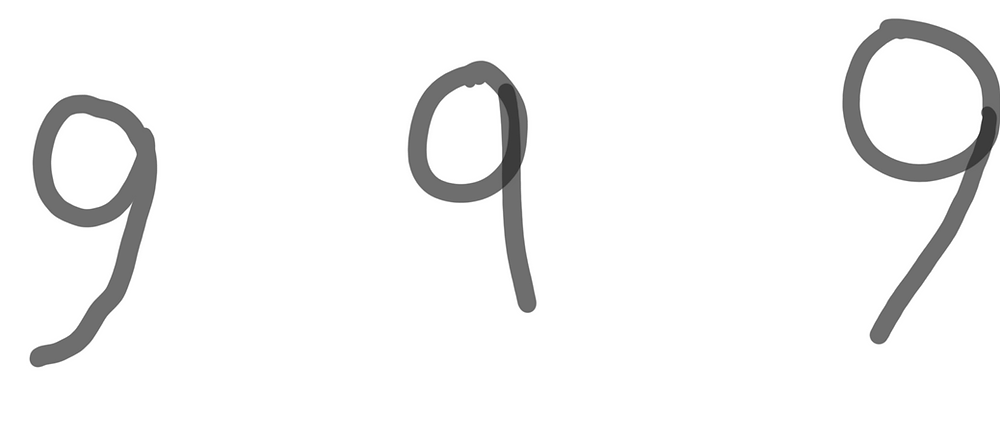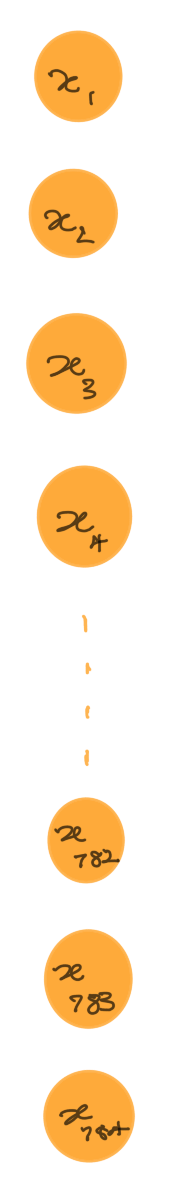Search
•Savidu Dias

# To Noobs with Love: Deep Learning

If you’ve stumbled on to this post, you’re most likely new to Deep Learning and are wondering what it is. As the title suggests, this is an article about Deep Learning for Noobs. I am fairly new to Deep Learning as well, and am writing this so that I can have a better understanding on the subject by processing it better. If you’re new to Deep Learning, then this post is just for you!

Enough chit chat. Let’s dive straight into it.

## What is Deep Learning?

You might be aware of Deep Learning at this point even though you may not be completely familiar with it. Deep learning is involved in many cool technological advancements we see today. Here are some popular applications to Deep Learning.

• Self-driving cars

• Facial Recognition

• Speech Recognition

• Optical Character Recognition (OCR) - Identifying hand written numbers and letters.

• Language translation

Many things that you have seen today are powered by Deep Learning. Google Translate, Apple’s Face ID, Tesla Autopilot are among these popular applications. Additionally, this is also used in medical fields such as diagnosing cancers, among many others.

Artificial Intelligence vs. Deep Learning

At this point, you may be rightfully wondering what Deep Learning has to do with Artificial Intelligence? Are they the same thing? They are, but you could consider it to be a part of Artificial Intelligence.

If we take a look at the chart below, we would notice that Deep Learning is a subset of Machine Learning, which itself is a subset of Artificial Intelligence.### Artificial Intelligence

Let’s talk about AI first. A short way to describe AI would be “getting machines to mimic human behaviour”. If you’re not familiar with the Turing Test, it is basically a test to see if a human could differentiate between the responses from a human and from a computer.

A human produces a set of written questions to a group of humans and a computer. Once the questions are answered, the human has to identify if the responses were from a human or computer. The computer passes the Turing test if the human cannot tell the computer responses apart from the other humans.

### Machine Learning

Machine learning is “data driven”. This means that it is all about training a bunch of data taken previously and predicting possible outcomes in the future, as well as trying to identify patterns that may be of interest. There are two types of approaches to machine learning.

1. Supervised Learning

2. Unsupervised Learning

In Supervised Learning, the provided dataset is labelled. This means that the computer is told what the right answers are, and the computer’s job is to figure out the correct answers for any future inputs. Algorithms like Linear Regression, and KNN are used for supervised regression or classification.

Unsupervised Learning is providing the machine with a set of data without any labels, and asking it to find patterns in the data set. This uses algorithms such as K-Means to find any patterns by clustering or associating the dataset provided.

Deep Learning

As I mentioned earlier, Deep Learning is a subset of Machine learning in the sense that it also has a set of learning algorithms. However, these algorithms are much more complex (and awesome), and are commonly known as Neural Networks.

## Neural Networks

What on Earth are Neural Networks. When I was first introduced to them, I was told that these are computer algorithms that imitate the behaviour of actual human neuron cells in the brain. Not a helpful answer is it?

None of these biological mumbo jumbo is really important here, but bear with me. The dendrites in neurons receive signals and transmit those signals to the cell body. The cell body processes these signals and decides if they should trigger signals to other neuron cells. If the body decides to do so, the axon triggers a chemical transmission to other cells.You may notice that the diagram above somewhat resembles a neuron cell. This is an oversimplified approach to explaining how neural networks work.

1. On the left, we have n neurons. Each neuron carries a data input.

2. Each data input is multiplied by a weight w along the arrow, This is also known as a synapse.

3. The big circle in the middle resembling the cell body calculates the sum of all inputs multiplied by their respective weights and adds another predetermined number called bias.

4. This value is then passed on to the activation function. What this means is that if the result from step 3 is greater than or equal to 0, then we get 1 as the output. If it is less than 0, we get 0 as the output.

5. The output is either 1 or 0.If we move the bias to the right side of the equation in the activation function like sum(wx) >= -b, then the value for -b is called the threshold value. Therefore, if the sum of weights is greater than the threshold value, the output becomes 1. If less than the threshold value, the output is 0.

What we have been dealing with here is called a Perceptron. A perceptron is generated as follows:

1. Inputs are fed into the perceptron.

2. Weights are multiplied to each input.

3. Summation and then add bias.

4. Activation function is applied.

5. Output is triggered.

Note that what we have used for the activation function here is known as a step function. There are many other more complex and interesting activation functions that we will run into in the future.

### Perceptron: An example

Does this still sound confusing? Let’s try to understand this with a very easy example. Let’s imagine that you are in the market for a new smartphone and want to decide if you should buy one.

For this, let’s consider MKBHD’s five pillars of purchasing a great smartphone.

1. Build Quality

2. Battery

3. Performance

4. Camera

5. Display

For each smartphone we will use x1, x2, x3 and so on as the input variables for these pillars and assign any value between 0 and 1 to each of them. Let’s assume that we are looking into a smartphone Model X.

Model X has a great camera and performance, so we may assign 0.9 to x3 and 0.95 to x4. Unfortunately, the phone’s amazing camera and processing capabilities come at a cost of high power consumption. As a result, its battery is not that great and we will assign x2 to 0.4. The phone also has a decent design and display so you’d assign both of them a value of 0.5.Now that we have the inputs ready, we can initialize the weights. Note that the larger the weight, the more influential the corresponding input is.

Let’s assume that I want a simple phone with a good camera, but we also want it to look good. So, we would say that the build quality, camera, and display are important. We will assign the weights w1 = 5, w4 = 4, and w5 = 5. Additionally, we don’t really care about the performance and battery, so we will assign w2 = 2 and w3 = 1.We will assume the threshold value is 10, which is equivalent for the bias value being -10. Let’s add up all the inputs multiplied by their relevant weights and add the bias value. In this case, the summation is as follows:According to our activation function (step function), since the summation value of 0.5 is greater than 0, our output is 1. Therefore, the perceptron determines that the smartphone Model X should be purchased.

Note that if we selected a threshold value greater than 10.5, our algorithm would determine that the smartphone Model X should not be purchased. As you may be aware of at this point, varying the weights and thresholds will result in different possible decision making models.

It is the job of our training algorithm to look at a bunch of data and assign suitable values for the weights and threshold. Thereafter, our miniature neural network can make a decision as to if we should purchase a new smartphone based on its specifications.

### Neural Networks: Intuition

Now that we have a solid grasp on Perceptrons, let’s take a look at Neural Networks. Simply put, a perceptron is a single layer of a neural network. A multi-layer perceptron is a neural network. A basic neural network would look a little something like this.(source: https://www.researchgate.net)
1. Each of the inputs we have in our case is fed to a neuron in the first layer of a neural network. This is also known as the input layer.

2. On the other end on the right side, we have the output layer with each neuron representing an output.

3. Between these two layers are hidden layers.

4. The information is transferred from one layer to another over connecting channels. Each of these connections have a value to them and therefore known as weighted channels.

5. All neurons in the hidden layers have their own associated bias values.

6. These biases are added to the weighted sum of inputs reaching each neuron which is then applied to the activation function.

7. The result of the activation function determines if the neuron gets activated. Every activated neuron passes on information to the next layer.

8. This continues until the final layer (output later), which will only activate one neuron.

### Neural Networks: An example

Now that I have bored you with trying to explain neural networks, let’s see an example to get an idea of how this might work. We’ll take a look at the classic example of people writing different characters. What we see below is how three different students have written the same character.Although you weren’t told what this character is, it’s very clear to us that this is the digit 9. Take a moment to realise how amazing the human brain is! Although the human brain can easily recognize the digits, how are we going to make a computer do the same? This is where neural networks come in.Unlike humans, computers see everything in pixels. Imagine the hand-written number 9 written in a 28px x 28px grid. Now this makes things a little easier. How would a trained neural network identify this digit? Our pixel grid amounts to a total of 784pixels.Each of the 784 pixels can be fed to the neural network through the input layer like we see here.The pixel information from the input layer is transferred to the second layer (first hidden layer) over connecting weighted channels. Each of these connections have a value to them.

All neurons in the first hidden layer have hidden values B1, B2, etc. associated with it. These values are the bias values. This bias is added to the weighted sum of all input values and applied to the activation function.Every activated neuron passes on information to the third layer (second hidden layer).The activated neurons in the third layer finally communicate with the neurons in the output layer. Only one neuron will be activated in the output layer, which will correspond to the hand-written digit.

In order for a neural network to function well, the weights and biases need to be adjusted continuously.

The following video from 3Blue1Brown does an amazing job at explaining this neural network in more detail. You should definitely take a look if you might still find any of this to be confusing.